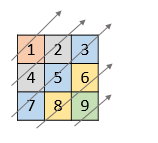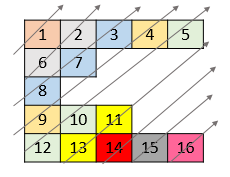# LeetCode 第186场周赛

### 第一题：分割字符串的最大得分

#### 题目描述

「分割字符串的得分」为 子字符串中 0 的数量加上 子字符串中 1 的数量。

• `2 <= s.length <= 500`
• 字符串 `s` 仅由字符 `'0'``'1'` 组成。

#### 题目链接

https://leetcode-cn.com/problems/maximum-score-after-splitting-a-string/

遍历每一个分割位置。

### 第二题：可获得的最大点数

#### 题目描述

• `1 <= cardPoints.length <= 10^5`
• `1 <= cardPoints[i] <= 10^4`
• `1 <= k <= cardPoints.length`

#### 题目链接

https://leetcode-cn.com/problems/maximum-points-you-can-obtain-from-cards/

#### 思路:

因为拿掉的牌固定为`k`张，因此剩下的就是中间的`n-k`张，维护一个滑动窗口，求中间`n-k`张的最小值即可。

### 第三题：对角线遍历 II

#### 题目描述• `1 <= nums.length <= 10^5`
• `1 <= nums[i].length <= 10^5`
• `1 <= nums[i][j] <= 10^9`
• `nums` 中最多有 `10^5` 个数字。

#### 题目链接

https://leetcode-cn.com/problems/diagonal-traverse-ii/

#### 思路:

在同一条对角线上的元素，横纵坐标之和是相等的，也就是`i+j`是一样的。

计算所有元素的横纵坐标，然后按照`(横纵坐标之和，纵坐标，元素值)`的关键字顺序来排序，排序后的结果就是对角线遍历的顺序。

### 第四题：带限制的子序列和

#### 题目描述

• `1 <= k <= nums.length <= 10^5`
• `-10^4 <= nums[i] <= 10^4`

#### 题目链接

https://leetcode-cn.com/problems/constrained-subset-sum/

#### 思路:

动态规划。令`dp[i]`表示选择下标为`i`的元素时能选择的最大子序和，因为选择的两个元素下标之差不能大于`k`，因此有状态转移方程`dp[i] = max(0, max(dp[i-k: i])) + nums[i]`

由于题目的范围较大，不能暴力计算`max(dp[i-k: i])`，因此使用一个最大堆来保存`dp[i-k: i]`的最大值。